Next: *CONTACT DAMPING Up: Input deck format Previous: *CONDUCTIVITY   Contents

## *CONSTRAINT

Keyword type: step

With *CONSTRAINT one can define constraints in a sensitivity step. It can only be used for design variables of type COORDINATE. Furthermore, exactly one objective function has to be defined within the same sensitivity step (using the *OBJECTIVE keyword).

A constraint is an inequality expressing a condition on the objective function. The inequality can be of type smaller than or equal'' (LE) or larger than or equal'' (GE). The reference value for the inequality is to be specified by a relative portion of an absolute value (the latter in the units used by the user). For instance, suppose the user introduces an absolute value of 20 and a relative value of 0.9 for a LE constraint on the mass. Than the mass is not allowed to exceed 0.9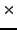20 = 18 mass units. If the absolute value is zero, the initial value is taken, e.g. for the mass this corresponds to the mass at the start of the calculation.

Right now, the following constraints are allowed:

• DISPLACEMENT: the sum of the square of the displacements in all nodes of the structure or of a subset if a node set is defined
• EIGENFREQUENCY: all eigenfrequencies calculated in a previous *FREQUENCY step
• MASS: mass of the total structure or of a subset if an element set is defined
• SHAPEENERGY: internal energy of the total structure or of a subset if an element set is defined
• STRESS: the maximum von Mises stress of the total structure or of a subset if a node set is defined. The maximum is approximated by the Kreisselmeier-Steinhauser function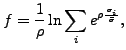(465)

where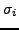is the von Mises stress in node i,and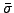are user-defined parameters. The higherthe closer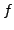is to the actual maximum (a value of 10 is recommended; the higher this value, the sharper the turns in the function).is the target stress, it should not be too far away from the actual maximum.

• THICKNESS: the maximum thickness across a node set w.r.t. an opposite node set.
• FIXGROWTH: the maximum thickness increase across a node set (only LE allowed).
• FIXSHRINKAGE: the maximum thickness decrease across a node set (only GE allowed).

First line:

• *CONSTRAINT.

Second line:

• the constraint
• an element or node set, if appropriate
• LE for smaller than or equal'', GE for larger than or equal''
• a relative value for the constraint, or, for the THICKNESS constraint, the opposite node set
• an absolute value for the constraint
•for the Kreisselmeier-Steinhauser function (only for the stress as constraint)
•for the Kreisselmeier-Steinhauser function (only for the stress as constraint)
Repeat this line if needed.

Example:

*CONSTRAINT.
MASS,E1,LE,,3.


specifies that the mass of element set E1 should not exceed 3 in the user's units.

Example files: .Next: *CONTACT DAMPING Up: Input deck format Previous: *CONDUCTIVITY   Contents
guido dhondt 2018-12-15